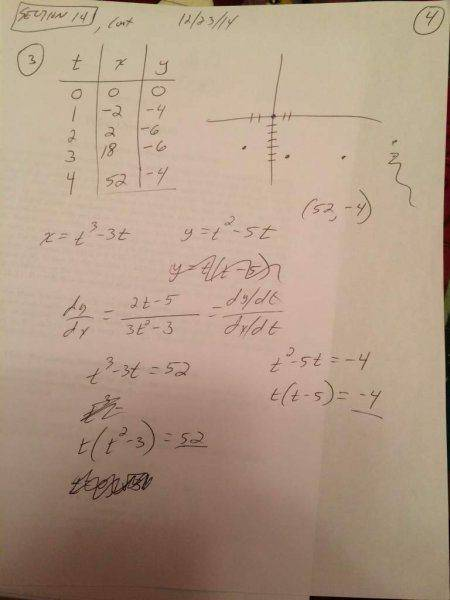# How do I find the tangent to this parametric curve?

Randall

## Homework Statement

Let C be the curve given parametrically by x = (t^3) - 3t; y = (t^2) - 5t
a) Find an equation for the line tangent to C at the point corresponding to t = 4
b) Determine the values of t where the tangent line is horizontal or vertical.

## Homework Equations

dy/dx = (dy/dt)/(dx/dt)
slope = d/dx of f(x,y)equation of a line: y-y1 = m (x-x1)

## The Attempt at a Solution

see attached - I know I need to solve for t, but I don't know how with these seemingly unsolvable equations because there is a t^3 and a t = 52 in the first equation and a t^2and a t = -4 in the second equation. Please help thanks!

Staff Emeritus
Homework Helper

## Homework Statement

Let C be the curve given parametrically by x = (t^3) - 3t; y = (t^2) - 5t
a) Find an equation for the line tangent to C at the point corresponding to t = 4
b) Determine the values of t where the tangent line is horizontal or vertical.

## Homework Equations

dy/dx = (dy/dt)/(dx/dt)
slope = d/dx of f(x,y) View attachment 76854
equation of a line: y-y1 = m (x-x1)

## The Attempt at a Solution

see attached - I know I need to solve for t, but I don't know how with these seemingly unsolvable equations because there is a t^3 and a t = 52 in the first equation and a t^2and a t = -4 in the second equation. Please help thanks!

It's not clear what your confusion is here. No, you don't need to solve for t; you are given the value of t for the point C on the curve. (Hint: t = 4)

For a) You have calculated an expression for dy/dx in terms of t. Use the given value of t to find the equation of the tangent line at C.

For b) For what value of m is the tangent line at C horizontal? For what value of m is the tangent line at C vertical? What are the values of t which give these two values of m?

Randall
I guess I'm not clear how to go about solving this problem then. Can you help me choose the correct procedure for finding the equation of the line tangent to the curve? Don't I have to take the derivative or something somehow? I'm not clear on what to do....

Staff Emeritus